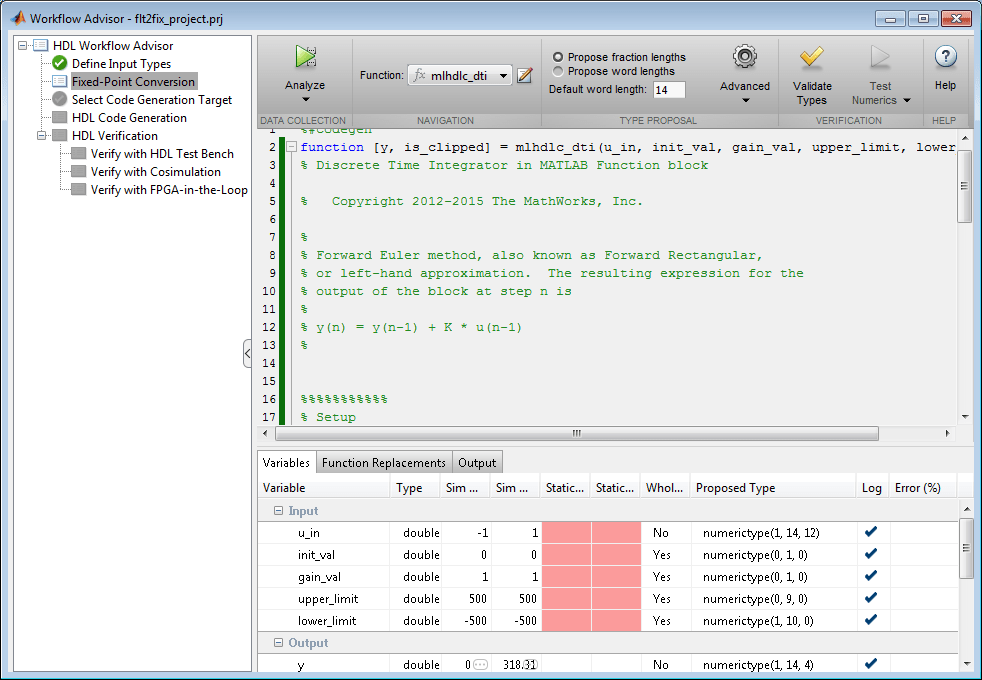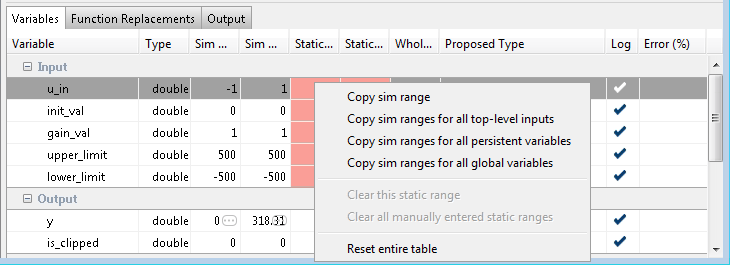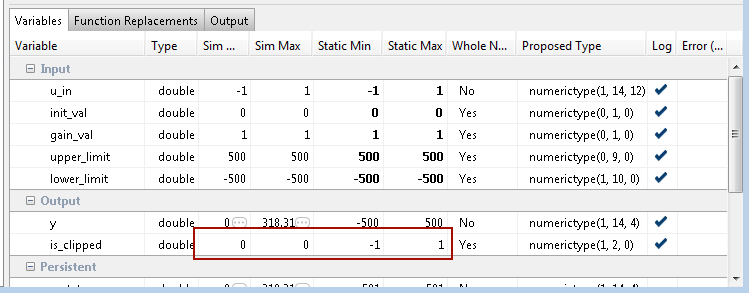# Fixed-Point Type Conversion and Derived Ranges

This example shows how to achieve your desired numerical accuracy when converting fixed-point MATLAB® code to floating-point code using static range analysis which helps to compute derived ranges of the variables from design ranges.

### Introduction

The floating-point to fixed-point conversion workflow in HDL Coder™ includes the following steps:

1. Verify the floating-point design is compatible for code generation.

2. Compute fixed-point types based on the simulation of the testbench.

3. Generate readable and traceable fixed-point MATLAB® code.

4. Verify the generated fixed-point design.

However, the fixed-point types proposed from the simulation depends on the quality of the testbench. Sometimes it is hard to write testbenches which completely cover paths of the design representing full design ranges of all the variables. Static analysis based workflow can be used in such cases to compute derived ranges from design ranges.

This tutorial uses a symmetric FIR filter whose output signal is integrated over time.

### MATLAB Design

The MATLAB code used in this example implements a simple Kalman filter. This example also contains a MATLAB testbench that exercises the filter.

```design_name = 'mlhdlc_dti'; testbench_name = 'mlhdlc_dti_tb'; ```
1. MATLAB Design: mlhdlc_dti

2. MATLAB testbench: mlhdlc_dti_tb

### Simulate the Design

Simulate the design with the testbench prior to code generation to make sure there are no runtime errors.

```mlhdlc_dti_tb ```### Create a New HDL Coder Project

To create a new project, enter the following command:

```coder -hdlcoder -new flt2fix_project_dmm ```

Next, add the file 'mlhdlc_dti.m' to the project as the MATLAB Function and 'mlhdlc_dti_tb.m' as the MATLAB Test Bench.

Refer to Get Started with MATLAB to HDL Workflow for a more complete tutorial on creating and populating MATLAB HDL Coder projects.

### Fixed-Point Code Generation Workflow

Perform the following tasks before moving on to the fixed-point type proposal step:

1. Click the 'Workflow Advisor' button to launch the HDL Workflow Advisor.

2. Choose 'Convert to fixed-point at build time' for the 'Fixed-point conversion' option.

3. Click 'Run' button to define input types for the design from the testbench.

4. Select the 'Fixed-Point Conversion' workflow step.

5. Click 'Analyze' to execute the instrumented floating-point simulation.

Refer to Floating-Point to Fixed-Point Conversion for a more complete tutorial on these steps.

### Determine the Initial Fixed Point Types

After instrumented floating-point simulation completes, you will see 'Fixed-Point Types are proposed' based on the simulation results.

At this step fixed-point types for each variable in the design based on the recorded min/max values of the floating point variables and user input.

Observe the simulation range of the variable 'is_clipped' in the function 'mlhdlc_dti'. You will notice that the simulation range of this variable is a constant value 0. However, if you can observe the code to see that the variable can take values from -1 to -1.

The ranges for the variable can be fixed by updating the testbench. However, it may be desirable to compute program ranges through static analysis.

### Entering Design Ranges and Computing Derived Ranges

In this step you can specify design ranges and compute derived ranges through static analysis. Enable derived range analysis by clicking the 'analyze ranges using derived range analysis' checkbox in the 'Analyze' button's menu. The tool will then prompt you to specify design ranges for the inputs variables in the Static Min and Static Max columns.There are multiple ways you can enter design ranges.

1. You can manually edit the 'Static Min' and 'Static Max' entries in the table and specify design ranges.

2. You can copy the Sim Min and Sim Max for a variable via right-clicking on the table cell (or)

3. You can Lock or Specify the Output type to be used as the design rangeOnce all the necessary design ranges are specified you can click on the 'Analyze' button to use derived range analysis.Notice that the derived range of the variable now includes values taken in all paths of the control flow.

### Insufficient design ranges

Sometimes specifying ranges for input variables alone may not be sufficient for certain designs. For example in a MATLAB design implementing a counter using a persistent variable, the range of the variable depends on number of times the design is called. In such situations you will see computed derived static ranges for the variable reported as -Inf or +Inf. When these imprecise ranges appear please consider specifying ranges for such persistent variables.